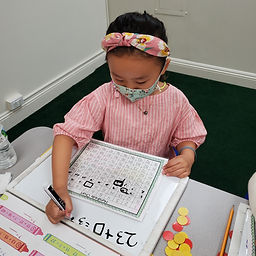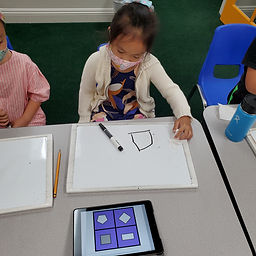## Ms. Raejean

### Target 1​

###### Lesson Type:

Continuation

Number Operation

:

Computation

Solve multi-step addition and subtraction problems within 50.

###### 1:

Solve problems that have more than one operation, addition and subtraction.

###### 2:

Understand when solving a problem that has both addition and subtraction operations that solving starts on the left and continues to the right.

2nd

###### Vocabulary:

Multi-step, Addition, Subtraction, Equation, Equal, Unknown Numbers

Activities:

Students continued to solve multi-step problems. Students solved

problems from left to right, then solved for the unknown number. First, students used counters to add or subtract a given amount. Then, students used hundreds charts to add and subtract. This allowed students to add or take away quantities with more ease.

Ex. ? + 17 + 14 -11 = 37

Step 1: 17 + 14 = 31

Step 2: 31 - 11 = 20

Step 3: ? + 20 = 37

Step 4: 37 - 20 = ?

The unknown number is 17.### Home Exploration

###### Guiding Questions:## Absent Students:

### Target 2

:

###### 1:

In a given group of objects, determine which objects does not fit with the others.

###### 2:

Name the property that objects have in common.

2nd

###### Vocabulary:

Classification, Properties

Activities:

Students identified the object that did not belong. Then students told why the object did not belong. Next, students told what the group of objects had in common. Different properties we identified are color, shape, and size.

Ex. Assortment of different sized and colored triangles, rectangles, and squares. The circle doesn't belong because it's round, and the other objects have corners.### Home Exploration

###### Guiding Questions:### Target 3

:

###### Vocabulary:

Activities:### Home Exploration